Problem title
Difficulty
Avg time to solve

Number of Longest Increasing Subsequence
Moderate
--
Longest String Chain
Moderate
--
Minimum ASCII Delete Sum for Two Strings
Moderate
--
Divisible Set
Moderate
--
Partition Array for Maximum Sum
Moderate
--
Eulerian Circuit
Hard
--
Find if Path Exists in Graph
Easy
45 mins
Critical Connections in a Network
Hard
--
Is Graph Bipartite?
Moderate
--
Valid Arrangement of Pairs
Hard
--

# Ninja and Binary Tree

Difficulty: MEDIUM
Avg. time to solve
25 min
Success Rate
75%

Problem Statement

#### For example: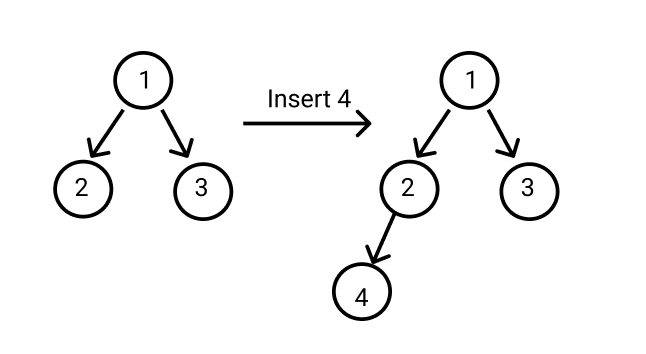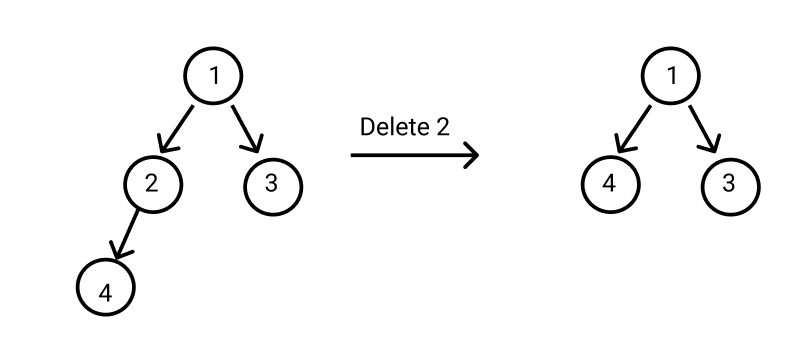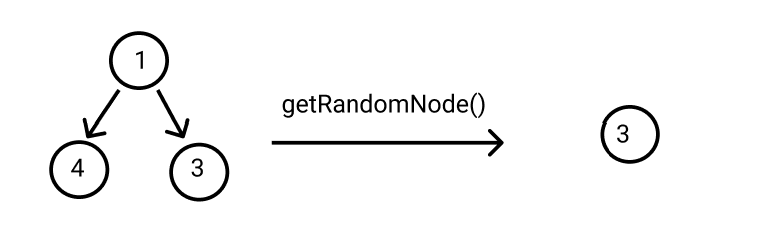##### Input Format
``````The first line of input contains an integer 'T' representing the number of test cases. Then the test cases follow.

The next line of each test case contains an integer ‘Q’ representing the number of queries to Ninja.

The next line, ‘Q’ lines of each test case, contain a character and integer representing which type of query is to perform on the binary tree and the value of the node.
``````
##### Output Format :
``````For each test case, return the value of the Random Node obtained from the get Random Node query.

Print the output of each test case in a separate line.
``````

#### Note:

``````You do not need to print anything; it has already been taken care of. Just implement the given function.
``````
##### Constraints :
``````1 <= ‘T’ <= 10
1 <= ‘Q’ <= 10000
Char = {‘I’, ‘D’, ‘R’}
1 <= ‘VAL’ <= 100000

Time Limit: 1 second
``````

#### Sample Input 1:

``````1
5
I 2
I 4
I 5
D 4
R
``````
##### Sample Output 1:
``````Valid answer
``````

#### Explanation for Sample Output 1:

``````For sample test case 1:
``````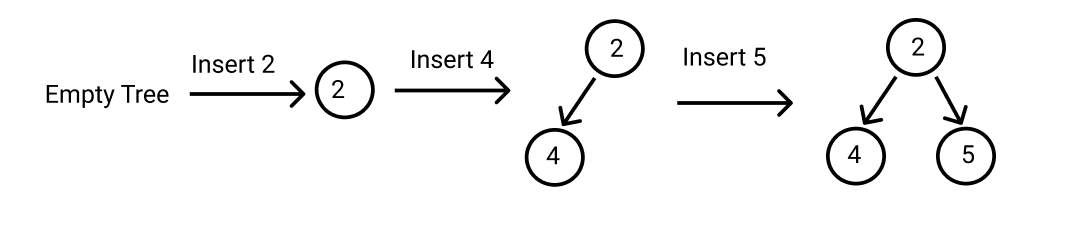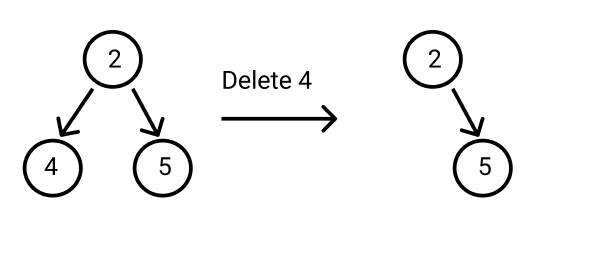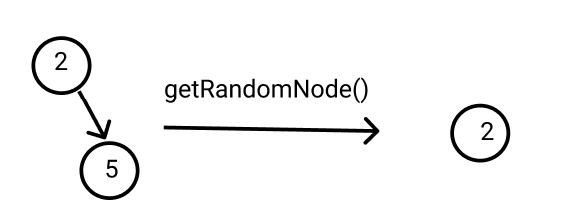``````In this sample test case, the random nodes may be 2 or 5 because all nodes should be equally likely to be chosen. So you can print any one of them.
As the random node returned (here 2) exists in the tree, the output is a “Valid answer.
``````
##### Sample Input 2:
``````1
5
I 4
I 2
D 2
I 5
R
``````
##### Sample Output 2:
``````Valid answer
``````

#### Explanation for Sample Output 2:

``````For sample test case 1:
``````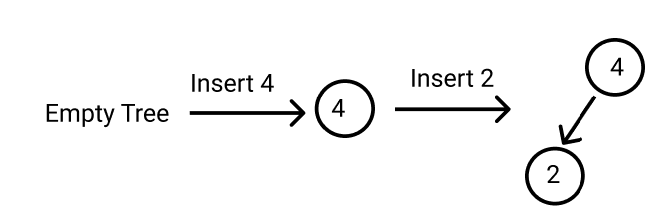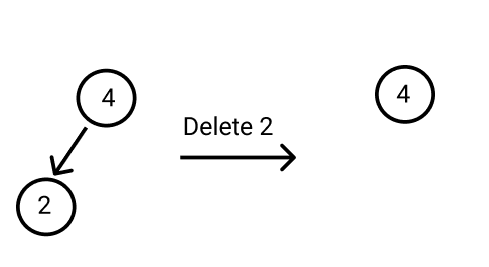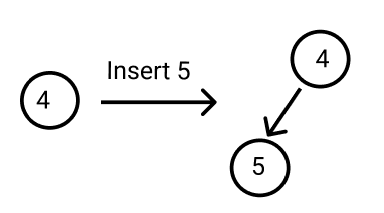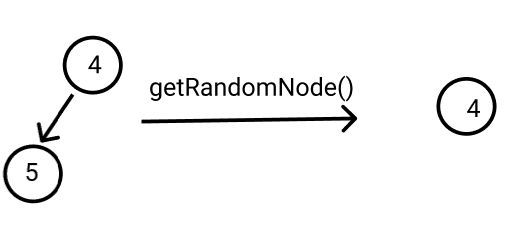``````In this sample test case, the random nodes may be 4 or 5 because all nodes should be equally likely to be chosen. So you can print any one of them.

As the random node returned (here 4) exists in the tree, the output is a “Valid answer”.
``````Console